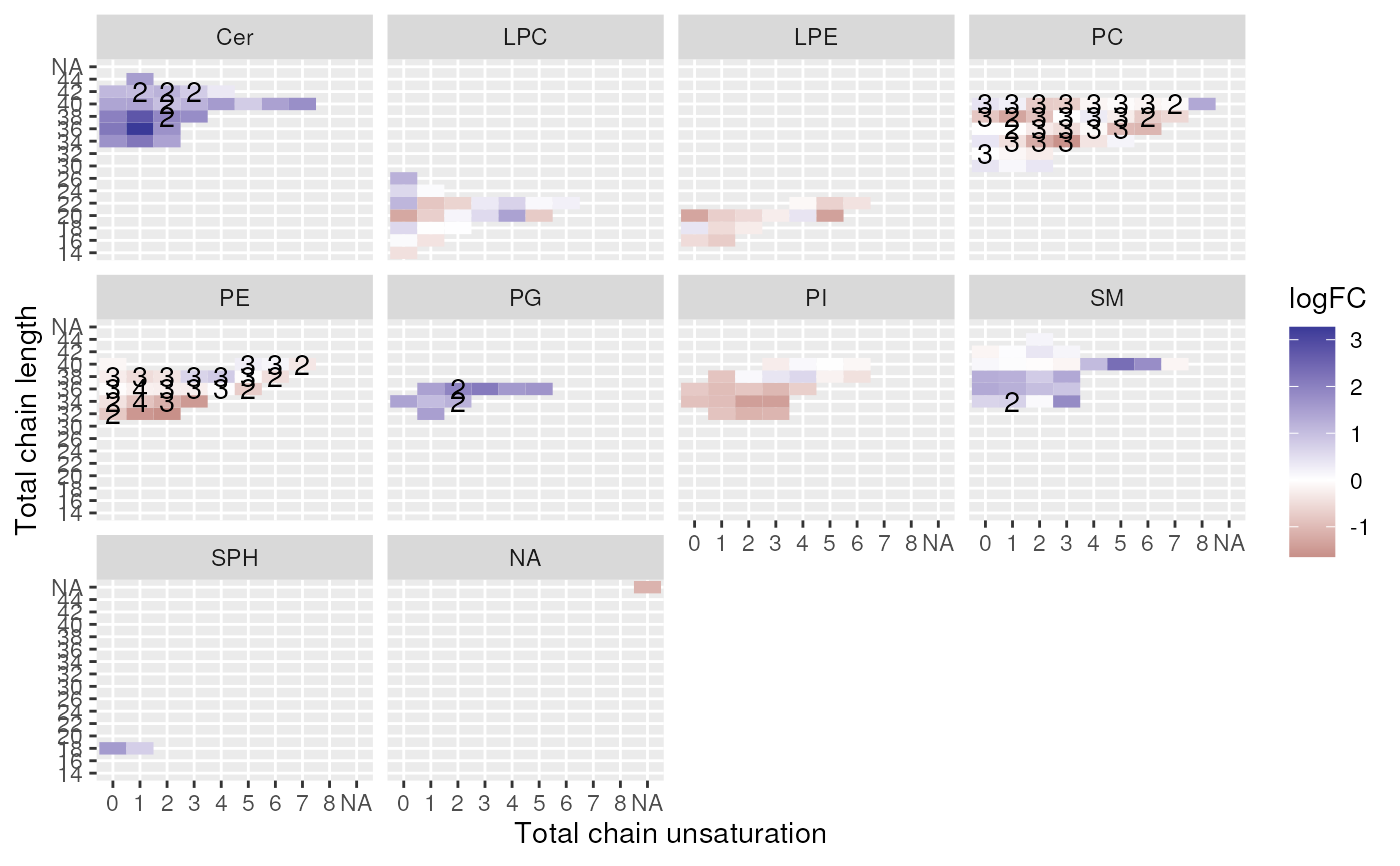Plot a chart of (log2) fold changes of lipids per class showing chain lengths and unsaturations. If multiple molecules with the same total chain length and unsaturation are present in the dataset, the measure is averaged, and the number of molecules is indicated on the plot.

plot_chain_distribution(de_results, contrast = NULL, measure = "logFC")

## Arguments

de_results

Output of de_analysis().

contrast

Which comparison to plot. if not provided, defaults to the the first comparison.

measure

Which measure to plot the distribution of: logFC, P.Value, Adj.P.Val. Default is logFC

A ggplot object.

## Examples

data(data_normalized)
de_results <- de_analysis(
data_normalized,
HighFat_water - NormalDiet_water,
measure = "Area"
)
plot_chain_distribution(de_results)